# Image-based skin color and texture analysis/synthesis by extracting

## Transcription

Image-based skin color and texture analysis/synthesis by extracting
```Image-based skin color and texture analysis/synthesis by extracting
hemoglobin and melanin information in the skin
Nobutoshi Ojima†
Kayoko Sato* Mitsuhiro Shiraishi†
Hideto Shimizu*
Norimichi Tsumura*
†
†
†
Syuuichi Akazaki
Kimihiko Hori
Yoichi Miyake*
Hirohide Nabeshima
*
Chiba Univerisity
Abstract
This paper proposes an E-cosmetic function for digital images
based on physics and physiologically-based image processing. A
practical skin color and texture analysis/synthesis technique is
introduced for this E-cosmetic function. Shading on the face is
removed by a simple color vector analysis in the optical density
domain as an inverse lighting technique. The image without
shading is analyzed by a previously introduced technique that
extracts hemoglobin and melanin components by independent
component analysis. Experimental results using UV-B irradiation
and the application of methyl nicotinate on the arms support the
physiological validity of the analysis and the effectiveness of the
proposed shading removal. We synthesized the way facial images
changed due to tanning or alcohol consumption, and compared the
synthesized images with images of actual changes in skin color.
The comparison shows an excellent match between the
synthesized and actual images of changes due to tanning and
alcohol consumption. We also proposed a technique to synthesize
the change of texture in pigment due to aging or the application of
cosmetics. The pyramid-based texture analysis/synthesis
technique was used for the spatial processing of texture. Using the
proposed technique, we could realistically change the skin color
and texture of a 50 year-old woman to that of a 20 year-old
woman.
CR Categories: I.3.3 [Computer Graphics] Picture/Image
Generation;
I.4.7 [Image Processing]: Feature Measurement;
I.4.10 [Image Processing]: Image Representation
Keywords: skin color, skin texture, melanin, hemoglobin, inverse
lighting, independent component analysis, pyramid-based texture
analysis and synthesis, physiologically-based rendering
1 Introduction
The reproduction of human skin color and texture may be
considered the most important function of various imaging
systems. With the recent progress of various imaging systems,
--------------------------------------------
†
Kao Corporation
such as mobile phones with CCD cameras, cosmetic advisory
systems [Ojima et al. 2002], and telemedicine systems, the
reproduction of skin color and texture has become increasingly
important for image communication, cosmetic recommendations,
medical diagnosis, and so on.
Reproduced skin color and texture depends on imaging
devices, illuminants and environments. As a result of the recent
progress of color management technology, imaging devices and
the color of an illuminant can be calibrated by device profiles (see
[ICC 1998, IEC 1999] for examples). High-fidelity reproduction
is not always effective in the practical imaging systems used for
facial imaging; therefore, additional functions for color and
texture reproduction [Hunt 1995] are required. These functions
can be labeled "E-cosmetic," "digital cosmetic," and also "Emake" [Numata et al. 1999].
Comparing the use of facial cosmetics in America and Europe,
and Asia, it seems that lipstick and makeup with some color is
generally preferred in Europe and America. In Asia, however, it
seems that more subtle or natural changes in skin color and
cosmetics for skin care purposes, such as whitening essence, are
generally preferred. In this paper, the "E-cosmetic" function
focuses on Asian people. The inverse lighting technique (see
[Marschner, and Greenberg 1997, Marschner 1998, Blanz and
Vetter 1999, Ramamoorthi and Hanrahan 2001] as examples) is
the key technology for E-cosmetic, because it is necessary to
obtain a unique reflectance on the skin by discounting illuminants.
E-cosmetic appropriately applies image-processing techniques
based on the original skin color.
With the help of computer graphics, computer vision and
image-processing techniques, skin color and texture reproduction
techniques are expected to be developed into the skin color and
texture analysis/synthesis techniques used for E-cosmetic. Here,
we will briefly review skin color and texture synthesis using
computer graphics and computer vision techniques. The
traditional technique for skin color synthesis is to map the skin
image on a mesh of the face. This texture-mapping technique is
very simple and easily implemented on computer hardware.
However, texture mapping and Phong shading are not enough to
represent accurate shading of skin color. Hanrahan and Krueger
 have modeled skin subsurface structure and photon
migration in the skin, and have simulated the shading of skin color.
Ishii et al.  also generated human skin texture, based on
optical scattering in the skin layer with a 3D mesh structure of the
skin surface. They reproduced the change in texture appearance
for various scattering coefficients in the skin. Jensen et al.
[2001,2002] reproduced very realistic facial images quickly,
based on photon mapping or the diffusion equation technique for
subsurface scattering. Skin color and texture will also change
according to the environmental illuminant. Debevec et al. 
proposed a technique to obtain the reflectance field of a face at
each point by rotating the light source around the face. They could
reproduce the facial image under an arbitrary environmental
illuminant, based on the obtained reflectance field. If it can be
Independent component
analysis with shading removal
Physiologically-based
image processing
Melanin
Body reflection
Original image
Synthesized image
Separation of body and
surface reflection
Hemoglobin
Shading
Surface reflection
Analysis
Process
Synthesis
Figure 1. Schematic flow of the imaging process in proposed image-based skin color and texture analysis/synthesis
assumed that the spectral reflectance property is homogeneous in
the facial skin area that is illuminated by a single illuminant at a
known position, an image-based technique can be used to get the
BRDF on the skin from a single digital image [Marschner 1998,
Marschner et al. 1999].
We believe that rendering techniques with subsurface
scattering can be used to realistically synthesize various skin color
images for E-cosmetic by applying a cosmetic layer on the skin
surface or changing the optical properties of the skin. In applying
this synthesis to the skin image taken by a digital camera, it is
necessary to analyze the skin image based on inverse lighting (see
[Marschner, and Greenberg 1997, Marschner 1998, Blanz and
Vetter 1999, Ramamoorthi and Hanrahan 2001] as examples) and
inverse scattering techniques [Tsumura et al. 2001] to obtain
model parameters, such as the depth of the skin layer, the
scattering coefficients and the densities of pigment. However, it is
a difficult, ill-conditioned problem to apply inverse lighting and
inverse scattering techniques to skin color imaging, since the
rendering techniques of subsurface scattering are modeled with
many parameters and nonlinear processing. The reflectance field
or BRDF at each point on the face may prove to be very useful for
inverse lighting and the inverse scattering techniques. However, to
obtain the information for inverse lighting problems, a special
image-acquiring system, or a homogeneous BRDF in the facial
skin area illuminated from a known single position, is required.
Also, the inverse scattering technique for the reflectance field or
BRDF has not yet been reported, as far as we know. It is our
expectation that, for practical use, E-cosmetic will use digital
images taken by a commercially-available digital camera and will
employ simple inverse lighting and scattering techniques.
Human skin is a turbid medium with a multi-layered structure.
Melanin and hemoglobin pigments are contained in this medium
[Gemert et al. 1989]. Slight changes of pigment construction in
the skin produce a rich variation of skin color. Freckles and suntan,
caused by melanin pigment, and the enhancement or suppression
of the appearance of blood circulation, caused by hemoglobin
pigment, are the major reasons that Asians apply cosmetics on
their faces. Therefore, as the first step of our physically and
physiologically-based E-cosmetic function, it is necessary to
analyze and synthesize skin color and texture on the basis of
hemoglobin and melanin pigment. Until now, a practical method
for this function has not been offered.
In this paper, we propose an E-cosmetic function for digital
images, based on physics and physiologically-based image
processing. A practical skin color and texture analysis/synthesis
technique is introduced for this function by extracting hemoglobin
and melanin information from a single skin color image taken by
digital camera. The proposed technique is based on the technique
reported by Tsumura et al. , in which hemoglobin and
melanin pigments are extracted from a single skin color image by
independent component analysis. In their technique, the scattering
in the skin was modeled in a simple linear form in the optical
density domain, and inverse optical scattering could be performed
by a simple inverse matrix operation. They also proposed the use
of extracted pigments to synthesize various skin colors by
changing the amount of pigment. In practical applications,
however, there is shading on the face caused by directional light,
which the previous (i.e., [Tsumura et al. 1999]) technique did not
address. Therefore, this technique leads to an incorrect estimate of
the pigment density in the shading area and cannot provide a
realistic synthesis for the entire area of the face. In this paper, we
model an imaging process, taking shading into consideration, and
we propose a technique to remove shading by simple inverse
lighting techniques that incorporate the previous pigment
extraction technique. To synthesize skin color in the previous (i.e.,
[Tsumura et al. 1999]) technique, a constant is simply multiplied
to the extracted amount of pigment. However, for a realistic
synthesis of skin color and texture, the amount of pigment should
be changed spatially with nonlinear processing, based on
physiological knowledge or a database containing the spatiotemporal variation of skin color and texture. In this paper, we also
propose a realistic skin texture synthesis technique for E-cosmetic
function, by changing the extracted amount of pigment spatially
using a single reference skin image as the target skin texture. A
pyramid-based texture analysis/synthesis technique [Heeger and
Bergen 1995] is used to change the amount of pigment spatially.
We also propose that the image, extracted by independent
component analysis, can be used as the initial image for
histogram-matching iterations in the pyramid-based synthesis.
The results demonstrate realistic modification of skin color and
texture.
Observed signals
Source signals
（Density of pigments）（Color vector）
a)
c1(x,y)
s1(x,y)
Independent
A
b)
s2
e1(x,y)
H
Independent
c2(x,y)
s2(x,y)
Mixture
c)
c2 a
s2
e2(x,y)
Separation
d)
2
a1
s1
c ( x, y ) = As( x, y ) .
e2
c
s1
The elements in the compound color vector indicate the pixel
values of the corresponding channels. Let us denote A = [a1 , a 2 ]
as the constant 2 × 2 mixing matrix, whose column vectors are the
pure color vectors, and s ( x, y ) = [ s1 ( x, y ), s2 ( x, y )]t as the
quantity vector on the image coordinates ( x, y ) . Equation (1) can
be rewritten in vector and matrix formulation as follows:
Extracted signals
c1
e1
Figure 2. Mixture and separation of independent signals
1.1 Organization of the paper
In Section 2, the technique of Tsumura et al., which
extracts hemoglobin and melanin pigments by independent
component analysis, is briefly reviewed. In Section 3, shading
removal by color vector space analysis is proposed as a simple
inverse lighting technique, and in Section 4, the effectiveness of
the technique is confirmed by practical experiments. In Section 5,
various synthesized skin color images are shown and compared to
real images. In Section 6, the technique for image-based skin
texture analysis/synthesis using physiologically-based image
processing, as is found in the pyramid-based technique, is
proposed and the effectiveness of this technique is also
demonstrated by experiments. Figure 1 shows the schematic of
flow in the proposed image-based skin color and texture
analysis/synthesis. The original image is separated into the images
of surface and body reflection, and the body reflection image is
analyzed by independent component analysis with the proposed
shading removal to obtain the melanin, hemoglobin, and shading
components. Physiologically-based image processing is applied
to the melanin and hemoglobin components. The processed
components are synthesized to obtain the image using E-cosmetic.
2 Image-based skin color analysis and synthesis
The previous technique [Tsumura et al. 1999] to extract
hemoglobin and melanin pigments from a single skin color image
is briefly reviewed in this section. Here, only two color channels
in the imaging device are considered and the simple additive
pigment mixing is used for ease of explanation. Note that
Tsumura et al.  actually used a more physically correct
multiplicative mixing model, treated additively in log color space,
with shortcomings that are overcome as described in the next
section
Let s1 ( x, y ) and s 2 ( x, y ) denote the quantities of the two
pigments, hemoglobin and melanin, which depend on the image
coordinates ( x, y ) in the digital color image. The pure color
vectors of the two pigments, are denoted a1 and a 2 , respectively,
per unit quantity. It is assumed that a1 and a 2 are different from
each other. It is also assumed that the compound color vector
c ( x, y ) on the image coordinates ( x, y ) can be calculated by the
linear combination of the pure color vectors with the quantities
s1 ( x, y ) and s2 ( x, y ) as
c ( x, y ) = s1 ( x, y )a1 + s2 ( x, y )a 2 .
(1)
(2)
In independent component analysis, it is also assumed that the
values s1 ( x, y ) and s2 ( x, y ) of the quantity vector are mutually
independent for the image coordinates ( x, y ) . Figure 2(a) shows
the process of mixing, and Figure 2(b) is an example of the
probability density distribution of s1 ( x, y ) and s2 ( x, y ) , which are
mutually independent. Figure 2(c) shows the probability density
distribution of c1 ( x, y ) and c2 ( x, y ) , which are elements of the
compound color vector c ( x, y ) . It is noted that the observed color
signals c1 ( x, y ) , c2 ( x, y ) are not mutually independent.
The relative quantity and pure color vectors of each pigment
can be extracted from the compound color vectors by independent
component analysis without a priori information about the
quantity and color vector. Let us define the following equation by
using the separation matrix H and the separated vector e ( x, y ) ,
as shown in Figure 2(a):
e ( x, y ) = Hc ( x, y ) ,
(3)
where
H = [ h1 , h2 ]
is
the
separation
matrix,
and
e ( x, y ) = [e1 ( x, y ), e2 ( x, y )]t is the extracted signal vector. By
finding the appropriate separation matrix H , we can extract the
mutually independent signals e1 ( x, y ) and e2 ( x, y ) from the
compound color vectors in the image. Many methods for finding
the separation matrix H have been proposed (see [Hyvarinen et
al. 2001] as an example). In this paper, optimization techniques
based on the fixed-point method [Hyvarinen and Oja 1997] are
used to find the separation matrix H .
Note that the extracted independent signals e1 ( x, y ) and
e2 ( x, y ) may actually correspond to s2 ( x, y ) and s1 ( x, y ) ,
respectively. Further, it is impossible to determine the absolute
quantities of s1 ( x, y ) and s2 ( x, y ) without additional assumptions.
In general, the extracted independent vector e ( x, y ) is given by
e ( x, y ) = RΛs ( x, y ) ,
(4)
where R is a permutation matrix that may substitute the elements
of the vectors for each other, and Λ is a diagonal matrix that
relates the absolute quantities to relative quantities. Substituting
Equations (2) and (3) into Equation (4), we obtain
HAs ( x, y ) = RΛs ( x, y ) .
(5)
Thus, the matrix HA should be equal to the matrix RΛ , and the
mixing matrix A is calculated by using the inverse matrix of H
as follows:
A = H −1 RΛ .
(6)
In practice, for our applications involving color image separation,
followed by editing of the separated images and resynthesis, the
permutation and scaling matrices do not influence the results, so
we set them to be identity matrices; i.e., we set A = H −1 .
Once we have obtained the mixing matrix A, we can perform an
analysis step on an image to extract the relative quantities s( x, y )
by applying A −1 to the observed signals c ( x, y ) . We can then
edit the relative quantities and synthesize a new image by
applying A to s ( x, y ) as in Equation (2).
If the number of pigments is larger than the number of
channels, it is difficult to extract the independent components by
relying on the reduction of signals. On the other hand, if the
number of pigments is smaller than the number of channels, it is
possible to make the number of channels equal to the number of
pigments by first finding the desired subspace using principal
component analysis. Tsumura et al.  use this technique to
Light source
Polarizers
Digital camera
Air
surface
reflection
body
reflection
Epidermis
Melanin
ρ m ,σ m (λ ), lm (λ )
ρ h ,σ h (λ ), lh (λ )
Dermis
Blood(Hemoglobin)
Figure 3. Schematic model of the imaging process
find a 2D hemoglobin-melanin color subspace given 3-channel
color data.
3 Shading removal by color vector space
analysis: simple inverse lighting technique
The previous technique leads to an inaccurate estimate of the
pigment densities, because it fails to address shading on the face
caused by directional light. Therefore, this technique could not be
applied to our practical application. In this section, the method for
shading removal by color vector space analysis is proposed as a
simple inverse lighting technique. The imaging process is
modeled first, as shown in Section 3.1, and based on this model,
the shading removal technique is described in Sections 3.2.
ci ( x, y ) = k ∫ L( x, y, λ ) si (λ ) dλ
(8)
= k ∫ exp{− ρ m ( x , y )σ m ( λ )l m ( λ ) − ρ h ( x , y )σ h ( λ )l h ( λ )}E ( x, y, λ ) si (λ ) dλ ,
where si (λ ) (i = R, G , B) is the spectral sensitivity of the digital
camera, and k is a constant value determined from the gain of the
camera. Research has shown that the spectral sensitivities of
digital cameras can, under some circumstances, be treated as
nearly orthogonal [Drew et al. 2002]. We take this to an extreme
and treat the sensitivities as delta functions, si (λ ) = δ (λ − λi ) .
(Later we relax this assumption.) In addition, we assume that the
lighting environment is distant and that its spectrum does not vary
with direction, so that the irradiance can be written as
E ( x, y, λ ) = p( x, y ) E (λ ) , where p( x, y ) encodes shape-induced
shading variation. Under these assumptions, Equation (8)
simplifies to:
ci ( x, y )
= k exp{− ρ m ( x , y )σ m ( λi )l m ( λi ) − ρ h ( x , y )σ h ( λi )lh ( λi )} p( x, y ) E (λi )
(9)
However, one cannot always assume that the lighting environment
contains a single spectrum; for example, when near a window,
room light and sunlight illuminate the face from different
directions. While we cannot account for multiple illuminant
sources using this technique, we can actively illuminate the face
with a camera flash, which creates a dominant single illuminant
color on the face because of its high radiance.
If we take the logarithm of Equation (9), we obtain the
following equation by vector and matrix formulation:
c log ( x, y ) = − ρ m ( x, y )σ m − ρ h ( x, y )σ h + p log ( x, y )1 + e log , (10)
where,
c log = [log(cR ( x, y )), log(cG ( x, y )), log(cB ( x, y ))] ,
t
3.1 Imaging model
Figure 3 shows the schematic model of the imaging process with a
two-layered skin model. We should note that melanin and
hemoglobin are predominantly found in the epidermal and dermal
layers, respectively. Part of the incident light is reflected on the
surface as a Fresnel reflection, and other parts penetrate into the
epidermis layer and dermis layers and are diffusely reflected from
the surface. The body reflection can be written as Equation (7),
assuming that the Lambert-Beer law is applicable in the skin layer
for incident light. In near-infrared light, it is reported that the
modified Lambert-Beer law [Hiraoka et al. 1993] is applicable by
using the mean path length of photons in the medium as the depth
of the medium (in the conventional Lambert-Beer law). The
modified Lambert-Beer law is assumed in Equation (7):
L( x, y, λ ) = exp{− ρ m ( x , y )σ m ( λ )l m ( λ ) − ρ h ( x , y )σ h ( λ )lh ( λ )}E ( x, y, λ ) (7)
where λ is the wavelength, E ( x, y, λ ) and L( x, y, λ ) are the
incident spectral irradiance and reflected spectral radiance,
respectively, at the position ( x, y ) on the surface, and
ρ m ( x, y ) , ρ h ( x, y ) , σ m (λ ) , σ h (λ ) are the pigment densities and
spectral cross-sections of melanin and hemoglobin, respectively.
For the modified Lambert-Beer law [Hiraoka et al. 1993], lm (λ )
and lh (λ ) are the mean path lengths of photons in the epidermis
and dermis layers, respectively,.
Surface reflection is removed by polarization filters in front of
the camera and light source by using the algorithm proposed by
Ojima et al. . The surface reflection is later added to the
synthesized images to obtain the final image. In principle, a colorspace separation technique (see [Klinker et al. 1990, Sato and
Ikeuchi 1994, Tominaga and Wandell 1989] as examples) for
surface and body reflection can be used for this purpose.
Therefore, the sensor response ci (i = R, G , B ) from the digital
camera can be obtained as follows:
σ m = [σ m (λR )lm (λR ),σ m (λG )lm (λG ),σ m (λB )lm (λB )] ,
t
σ h = [σ h (λR )lh (λR ),σ h (λG )lh (λG ),σ h (λB )lh (λB )] ,
t
1 = [1, 1, 1] ,
t
e log = [log( E (λ R )), log( E (λ G )), log( E (λ B ))] ,
t
p ( x, y ) = log( p ( x, y )) + log(k ) ,
log
are used to write Equation (10) in simple terms. Therefore, the
observed signals v log can be represented by the weighted linear
combination of the three vectors σ m , σ h ,1 with the bias vector
e log , as shown in Figure 4. Equation (10) also indicates that
optical scattering in the skin is simply modeled linearly, and the
inverse scattering technique becomes a simple inverse matrix
operation.
The assumption that spectral sensitivity is narrow-band was
used to obtain Equation (10) easily. Here, we attempt to relax the
narrow-band assumption. We can decompose the wavelengthdependent hemoglobin and melanin scattering terms into constant
bias terms with wavelength-dependent residuals:
σ m (λ )lm (λ ) = σ m , ilm , i + σ m′ , i (λ )lm′ , i (π )
(11)
σ h (λ )lh (λ ) = σ h ,ilh ,i + σ h′ ,i (λ )lh′ ,i (π )
(12)
where bar and prime notation are used for the constant and
wavelength-dependent terms, respectively, and the i subscript
indicates that the decomposition is tuned for the i-th camera
spectral sensitivity. Substituting into Equation (8), we obtain:
ci ( x, y ) = k exp{− ρ m ( x , y )σ m,i lm,i
where
− ρ h ( x , y )σ h ,i l h ,i } p ( x, y ) mi ( x, y )
(13)
−cBlog
−1
2D plane extracted by PCA
c log ( x, y )
Strength
of shading
σm
Strength − 1
of shading
−1
σm
σh
σh
e log
σm
(a) without shading
−cRlog
θ
Rotate
σh
(b) with shading
Figure 5. Projection onto the skin color plane to remove shading
−cGlog
Figure 4. Observed signal, represented by the weighted linear
combination of three vectors with a bias vector
mi ( x, y ) =
∫ exp{− ρ m ( x , y )σ m′ ,i ( λ )lm′ ,i ( λ ) − ρ h ( x , y )σ h′ ,i ( λ )lh′ ,i ( λ )}E (λ ) si (λ ) dλ
(14)
Next, we make the assumption that mi ( x, y ) is approximately
constant over the image, i.e., mi ( x, y ) ≈ mi . This assumption is
reasonable, for instance, if σ m ( λ )lm ( λ ) and σ h ( λ )lh ( λ ) are smoothly
varying with wavelength, so that the residuals σ m′ ,i ( λ )lm′ ,i ( λ ) and
σ h′ ,i ( λ ) l h′ ,i ( λ ) are very small. The results of an experiment
performed in Section 4 suggest that the constancy assumption
described here holds reasonably well for real images of human
skin.
Finally, if we take the logarithm of Equation (14), we find
that Equation (10) still holds, but with different definitions of
terms as follows:
[
= [σ
]t
σ m = σ m , R l m , R , σ m ,G l m ,G , σ m , B l m , B ,
σh
l
h, R h, R
]t
, σ h ,G l h ,G , σ h , B l h , B ,
e log = [log(m R ), log(mG ), log(m B )] .
t
Let us now discuss the imaging model in Equation (10) in
detail. The vectors σ m , σ h are relative absorbance vectors for the
melanin and hemoglobin components, respectively. They are not
affected by the illuminant and the white balance of the camera,
but they may be affected by the depth of the epidermis and dermis
layers, since the mean path length changes with depth. In the color
vector space in Figure 4, the strength of the shading on surface
p log ( x, y ) is directed into the vector 1 at any position, and the
color of the illuminant is a constant vector e log at any position.
These two details show the illuminant-independent property of the
proposed analysis, and are used in Section 3.3 to remove the
shading on the face.
If the irradiance onto the surface is a constant
p log ( x, y ) = constant in all pixels of the analyzed skin area, we
can apply the independent component analysis to the 2D plane
spanned by the hemoglobin and melanin vectors σ m , σ h , as
written in Section 2 and shown in Figure 5(a). The 2D plane can
be obtained by principal component analysis (PCA), as performed
by Tsumura et al. .
3.2 Finding the skin color plane in the face
In practical application, shading on the face from directional light
leads to an incorrect estimate for the density of pigment on the
shaded area, as shown in Figures 7(a), (b) and (c). Figure 7(a) is
the original image of skin, and (b) and (c) are the extracted
melanin and hemoglobin pigments, respectively. It should be
noted that the shading caused by the nose produces a false
pigment estimate, which you can see in Figures 7(b) and (c).
Figure 5(b) shows the influence of shading in the color vector
space for the local area on the face. Since the texture of the skin’s
color is homogeneous in the local area, the strength of shading is
added to each value of skin color in 3D configuration. If we apply
the principal component analysis to the skin color that is
influenced by shading in the color vector space, the extracted 2D
plane will be rotated from an accurate plane to the direction of
vector 1 , as shown in Figure 5(b).We propose a procedure to find
the accurate skin color plane from the facial image. Based on the
resulting skin color plane, shading is removed from all pixels of
the skin in the entire face. Therefore, the accurate density of
pigment is extracted from all pixels without the undesirable
effects of the shading factor. The procedure to find the accurate
skin color plane is as follows.
Step 1. Prepare the facial image taken by digital camera.
Step 2. Set the window size N × M as a local area
for independent component analysis.
Step 3. Set the scanning start point for the analysis of the face.
Step 4. Determine the angle θ between the extracted skin
color plane by PCA and the vector 1 . If this is not
acceptable, go to Step 6. If acceptable, go to Step 5.
Step 5. Determine the extracted relative absorbance vectors σ m ,
σ h obtained by independent component analysis. If these
are not acceptable, go to Step 6. If acceptable, go to Step 7.
Step 6. Shift the window for analysis, and go back to Step 4.
Step 7. The obtained absorbance vectors σ m , σ h are the
analysis result for the face.
In Step 3, the scanning start point is set manually. In Step 4, the
plane extracted by PCA is examined by the angle θ between the
skin color plane extracted by PCA and the vector 1 . As shown in
Figure 5(b), if the strength of shading is not constant, the angle θ
is smaller than the one obtained from the accurate plane. We can
set the threshold value for the angle from a database of faces. A
total of 36 female facial images were used as the database for the
following experiment. Several windows were extracted manually
from each face in the database and analyzed with independent
component analysis. The extracted images are judged manually
for successful separation of the hemoglobin and melanin
components. It was possible to make these manual judgments in
areas with freckles or pimples. Based on the above samples, the
threshold value for the angle is determined by using a linear
discrimination function [Duda and Hart 1973].
Projection
−1
Strength
of shading
σm
σh
(a) Original image
(b) Melanin
(c) Hemoglobin
Conventional technique
2D plane extracted by PCA
in the proposed procedure
Figure 6. Projection onto the skin color plane to remove shading
In Step 5, the relative absorbance vectors σ m , σ h , are
extracted by independent component analysis and examined,
because sometimes the extraction fails to find the local minimum
in the optimization process for matrix H in Equation (3). This
may be caused by the influence of noise, and/or violation of the
independence assumption for some unusually pigmented areas.
The relative absorbance vectors σ m , σ h are illuminantindependent. This is shown in Equation (10). Based on the face
database that was used above, we confirmed that the absorbance
vectors vary within a restricted range for typical human faces.
Thus, we determine the amount of acceptable variation using a
linear discrimination function [Duda and Hart 1973] based on the
samples used above. In Step 5, an incorrect estimate rarely falls
within the restricted variation. In Step 7, the obtained absorbance
vectors σ m , σ h are the analysis result. We repeat the analysis
from Steps 1 through 7 several times to get stable absorbance
vectors.
3.3 Projection technique for shading removal
(d) Original image
(e) Melanin
Proposed technique
(f) Hemoglobin
Figure 7. The results of independent component analysis: (a),(d)
original image, (b),(e)melanin, (c),(f) hemoglobin by the
conventional technique (b)(c), and by the proposed technique
(e)(f)
(a) UV-B irradiation
(d) Methyl nicotinate application
By using the above procedure, we can find the appropriate 2D
skin color plane spanned by the absorbance vectors σ m , σ h .
Since the strength of shading is directed to the vector 1 for any
device and illuminant, the observed skin color vector can de
decomposed as follows.
c log = [σ m
[
]t
1] − ρ m ,− ρ h , p log + e log
σh
(15)
(b) Melanin
(e) Melanin
(c) Hemoglobin
(f) Hemoglobin
Rearranging, we arrive at:,
[− ρ
m
]t
,− ρ h , p log = [σ m
σh
1] (c log − e log )
−1
The bias vector e log is unknown. Therefore, if we assume that the
smallest value of each pigment in the skin image is zero, then e log
is calculated by eilog = min (vilog ( x, y )) for each band of color.
x, y
Based on the above decomposition, the shading term w p 1 is
removed as follows:
c log
projection = [σ m
σh
0 ][σ m
σh
1] (c log − e log ) + e log .
−1
(16)
This process is shown in Figure 6 in color vector space. Figures
7(d), (e) and (f) show the results of the proposed method. Figure
7(d) is the original image of the skin, and (e) and (f) are the
extracted melanin and hemoglobin pigments, respectively, with
the proposed shading removal. It should be noted that the shading
caused by the nose cannot be observed in Figures 7(e) and (f). We
conclude that the results of the proposed method, shown in
Figures 7(e) and (f), improves the analysis significantly compared
to the results of the conventional method, shown in Figures 7(b)
and (c).
Figure 8. UV-B irradiation: (a) original, (b) melanin, (c)
hemoglobin, Application of methyl nicotinate: (d) original, (e)
melanin, (f) hemoglobin
4 Experiments
In this section, the physiological validity of the proposed analysis
is confirmed by practical experiments. The arm of a subject is
irradiated by UV-B for the melanin component, and methyl
nicotinate (1 mg/ml solution) is applied to another arm for the
hemoglobin component, as methyl nicotinate is known to increase
the hemoglobin. An image of the arm, where UV-B (1.5
Minimum Erythema Dose) was irradiated in local rectangular
Hemoglobin
Melanin
Figure 9. Skin color synthesis with the change in pigmentation using the proposed shading removal technique for analysis. The center
image is the image of the original skin color.
(a) Original image
(b) Synthesized image
(c) Real wine drinking image
Figure 10. Alcohol consumption: (a) original image (b)
synthesized image (c) real image after alcohol consumption
(a) Original suntan image
(b) Synthesized image
(c) Real image before suntan
Figure 11. Tanning: (a) original tanning image (b) synthesized
image (c) real image before tanning
areas, was taken after two weeks with a digital camera (Nikon D1,
2,000 by 1,312 pixels). An image of the arm, where methyl
nicotinate was applied locally in a circular area,, was also taken
with a digital camera after 30 minutes of application. These
images were analyzed by the proposed method with a window
size of 100 by 100 pixels for both melanin and hemoglobin
components. Figure 8(b) shows the square patterns caused by
melanin, which indicate the biological response of melanin to UV
radiation. Figures 8(a), (b), and (c) show the original skin image
and the images of the densities for the melanin and hemoglobin
components, respectively. On the other hand, Figures 8(d), (e),
and (f) show the original skin image for methyl nicotinate and the
images of the densities for the melanin and hemoglobin
components, respectively. Figure 8(f) also shows the round
patterns, which indicate the biological response of hemoglobin to
methyl nicotinate. On the other hand, the patterns didn't appear in
the hemoglobin components in Figure 8(c) and melanin
components in Figure 8(e). It should also be noted that shading in
the lower part of the arm is removed, and the density of pigment is
extracted effectively from the entire area of the arm. These results
are valid physiologically, and show the effectiveness of the
proposed method of skin color image analysis. We can also
indirectly conclude that the approximation for the imaging model
with modified Lambert Beer law in Section 3.1 is also reasonable
for our applications.
5 Facial color image synthesis
A woman’s facial image is analyzed, and various facial color
images are synthesized realistically by changing the amount of
hemoglobin and melanin components. The results are discussed in
Section 5.1. Changes in facial color due to alcohol consumption
and tanning are also synthesized realistically, and the synthesized
images are compared with real images in Section 5.2.
5.1 Various skin color synthesis
Figure 9 shows examples of a realistic synthesis of a woman’s
face by uniformly decreasing or increasing the hemoglobin and
melanin components for every pixel in the image. The center
image is the image of the original skin color. Each column of
images indicates an increase or a decrease in the amount of
hemoglobin. From bottom to top, the amount of hemoglobin is
decreased or increased by adding a value of –0.2, -0.1, 0, 0.1, 0.2.
These values are relative values since the absorbance vector is
normalized in the process. Each row of images indicates an
increase or decrease in the amount of melanin. From left to right,
the amount of melanin is decreased or increased by adding a value
of –0.3, -0.1, 0, 0.1, 0.3. We can see very realistic changes in
facial color. It should be noted that the operator did not need to
have any knowledge of the skin color; the operator just changed
the amount of pigment. It is also noted that the synthesized images
are physiologically realistic, as explained in the previous section.
5.2 Alcohol consumption and tanning
Figures 10(a) and (b) and Figures 11(a) and (b) show the original
images and the images that were realistically synthesized by
scaling the hemoglobin and melanin components. Figures 10(c)
and Figure 11(c) show the real image after alcohol consumption
and before tanning, respectively. The images are synthesized by
uniformly scaling the amount of pigment, and the scale is adjusted
to match with the real image. By comparing Figures 10(b) and (c),
we can conclude that the proposed method can realistically
synthesize the change of hemoglobin due to alcohol consumption.
By comparing Figures 11(b) and (c), we can conclude that the
proposed method can realistically model the change of melanin
due to tanning.
6 Facial color image synthesis
For the realistic synthesis of beautiful skin color and texture, the
amount of pigment should be changed spatially with nonlinear
processing. In this section we took the skin image of a 50 yearold woman, analyzed and processed it, and converted it into a
synthesized skin image of a 20 year-old woman.
At first, we prepared skin images of 50 and 20 year-old
women. The women are mother and daughter; and were paired to
suppress all impressions other than the color and texture of
pigment as much as possible. Figures 12(a) and (c) shows the
original skin images for mother and daughter, respectively. The
extracted melanin images are analyzed by the pyramid-based
technique [Heeger and Bergeny 1995]. A Laplacian pyramid,
rather than a steerable filter pyramid, is used for the analysis and
synthesis of texture in this paper, since there were no unique
orientations in the distribution of pigment. The skin region is
manually segmented away from the eyes and then processed into a
Laplacian pyramid. The eye region is filled using the average
value of pigment around the eyes in order to construct the
Laplacian pyramid. The analyzed histograms in each level of the
pyramid for the 50 year-old woman are matched to the
histograms at the same level of the pyramid derived from the
image of the 20 year-old woman. For the target image of the 20
year-old woman, only a patch of the skin is used, which is shown
in Figure 13(c). Figure 13(a) show the original melanin texture of
50 year-old woman, and (b) shows the synthesized melanin
texture obtained from the proposed analysis and synthesis for the
original melanin texture of the 50 year-old woman, and (c) shows
the melanin texture of the 20 year-old woman used as the target
texture. These figures show that the original melanin texture of
the 50 year-old woman has been changed to that of the 20 yearold woman while maintaining the original distribution of pigments.
Figure 12(a) is the original image of the 50 year-old woman, and
12(b) is the same image of the 50 year-old woman after being
synthesized using the proposed technique. This shows very
realistic color and texture changes in the skin images. From the
skin image in Figure 12(b), we can conclude that the proposed
technique can be used as a very realistic E-cosmetic function.
7 Conclusion and discussion
Using the proposed practical skin color analysis/synthesis
(a) Mother
(b) Synthesized image
(c) Daughter
Figure 12. Skin color and texture synthesis for simulating cosmetic application: (a) original skin image of a 50 year-old woman, (b)
synthesized image from the proposed analysis and process of the original image (a), (c) original skin image of a 20 year-old woman.
(a) Mother
(b) Synthesized image
(c) Daughter
Figure 13. Skin texture synthesis for melanin: original melanin
texture of 50 year-old woman, (b) synthesized melanin texture
from the proposed analysis and process of the original image (a),
(c) original melanin texture of a 20 year-old woman used as a
target texture for the processing.
technique, we were able to synthesize extremely realistic images
showing the effects of alcohol consumption and tanning on skin
color. With the incorporation of the pyramid-based texture
synthesis/analysis technique, we could synthesize the realistic
change of texture and color image for skin, based on a database
for physiological matching. In the experiment in this paper, we
only used one target image in the database. The aging of skin
color and texture differs from one individual to another. Therefore,
it is necessary to further analyze the histograms at each level of
the Laplacian pyramid in the skin color and texture database to
reveal trends and to synthesize images with further physiological
validity.
For the simple analysis of skin images without ill-conditioned
problems, we approximated the imaging process as a simple
weighted combination of vectors. Our results in Section 4 show
the approximation was valid in our application. Because we have
the strict approximation form of Equation (11), an evaluation of
Equation (11) will prove the validity and accuracy of the proposed
analysis. Furthermore, the bias vector e log is obtained from the
assumption that the smallest value of each pigment in the skin
image is zero. Actually, this is not true, but this does not affect the
analysis of skin pigment density because the density obtained is a
relative value. However, in the synthesis of skin color and texture
using the target skin image, discussed in Section 6, the difference
of the bias vector causes a change of the mean skin color.
Therefore, we matched the histograms between the target image
and the synthesized image in optical density space to compensate
for this difference of bias color. For scattering in the skin, we
performed a linear approximation, based on the modified
Lambert-Beer law. By using this linear model and independent
component analysis, we could easily perform inverse optical
scattering without a priori information of the absorption
coefficient and mean path length, which is directed by the
scattering coefficient. However, spatial transport of light through
subsurface scattering cannot be represented by our method
because we modeled the imaging process at each pixel
independently. It is necessary to consider the dependence of pixels
for synthesizing the change of skin translucency.
Physiological reality is more complex in skin [Freefberg et al.
1999. ] Hemoglobin has two states: oxy-hemoglobin and deoxyhemoglobin. The two states have different spectral absorbances,
and the ratio between them will change spatially on the image of a
large area of skin or an area with skin diseases. It is also known
that there are at least two common varieties of melanin. There are
also other pigments in the skin. These factors will lead to some
errors in the analysis of skin images when using the proposed
technique. The proposed technique has not reached the stage
necessary for quantitative evaluation. Our technique has only been
verified by the resultant realistic images. Further research should
be conducted to clarify the limitations and robustness of the
proposed techniques.
8 Acknowledgements
We would like to thank the SIGGRAPH reviewers, particularly
the final revision referee, for helpful comments on the manuscript.
References
BLANZ V. AND VETTER T. 1999. A morphable model for the
synthesis of 3D faces. In Proceedings of ACM SIGGRAPH'99,
187-194.
DEBEVEC P., HAWKINS T., TCHOU C., DUIKER H.-P.,
SAROKIN W., AND SAGAR M. 2000. Acquiring the
reflectance field of a human face. In Proceedings of
SIGGRAPH 2000, 145-156.
DREW M. S., CHEN C., HORDLEY S. D., AND FINLAYSON G,
D. 2002. Sensor transforms for invariant image enhancement.
In Proceeding of IS&T /SID's Tenth Color Imaging Conference,
IS&T and SID, 325-330.
DUDA R.O. AND HART, P.E. 1973. Pattern Classification and
Scene Analysis. Wiley & Sons, Inc.
FREEFBERG I. M., EISEN A. Z., WOLFF K., AUSTEN F. K,
GOLDSMITH L.A., KATZ S. I., AND FITSPATRICK T. B.
1999 Fitzpatrick's Dermatology in General Medicine, McGraw
Hill.
GEMERT M. J. C. V, JACQUES S. L., STERBORG H. J. C. M,
AND STAR W. M. 1989. Skin Optics. IEEE Trans. Biomed.
Eng., 36 1146-1154.
HANRAHAN P. AND KRUEGER W. 1993. Reflection from
layered surfaces due to subsurface scattering. In Proceedings of
ACM SIGGRAPH '93, ACM Press / Computer Graphics
Proceedings, Annual Conference Series, ACM, 187-194.
HEEGER D. J. AND BERGEN J. R. 1995. Pyramid-based texture
analysis/synthesis. In Proceedings of SIGGRAPH 95, 229-238.
HIRAOKA M., FIRBANK M., ESSENPREIS M., COPE M.,
ARRIGE S. R., ZEE P. V. D., AND DELPY D. T. 1993. A
Monte Carlo investigation of optical pathlength in
inhomogeneous tissue and its application to near-infrared
spectroscopy. Phys. Med. Biol. 38, 1859-1876.
HUNT, R. W. G. H. 1995. The Reproduction of Colour, Fifth
edition, Fountain Press.
HYVARINEN A., KARHUNEN J., AND OJA E. 2001.
Independent Component Analysis, Wiley Inter-Science.
HYVARINEN A. AND OJA E. 1997. A fast fixed-point algorithm
for independent component analysis. Neural Computation 9,
1483-1492.
ICC (International Color Consortium) 1998. Spec ICC.1:1998-09,
File Format for Color Profiles. ICC.
JENSEN H. W., MAESHNER S. R., LEVOY M., AND
HANRAHAN P. 2001. A practical model of subsurface light
transport. In Proceedings of ACM SIGGRAPH 2001, 551-518.
JENSEN H. W. AND BUHLER J. 2002. A rapid hierarchical
rendering technique for translucent materials. In Proceedings of
SIGGRAPH 2002 576-581.
KLINKER G. J., SHAFER S.A. AND KANADE T. 1990. A
Physical Approach to Color Image Understanding",
International Journal on Computer Vision, 4(1) 7-38.
MARSHNER S. R. AND GREENBERG D. P. 1997. Inverse
lighting for photography. In Proceeding of Fifth IS&T/SID
Color Imaging Conference , IS&T and SID, 262-265.
MARSHNER S. R. 1998. Inverse Rendering for Computer
Graphics. Ph.D. thesis, Cornell.
MARSCHNER S. R., WESTIN S. H., LAFORTUNE E. P. F.,
TORRANCE K. E., AND GREENBERG D. P. 1999. Imagebased BRDF measurement including human skin. In
Proceedings of 10th Eurographics Workshop on Rendering,
139-152.
NUMATA K., RI K., AND KIRA K., 1999. "E-Make"; A real-time
HD skin-make-up machine. IEICE Technical report 23, 76, 1116.
http://www.nhk.or.jp/strl/open2001/en/tenji/id17/index.html.
OJIMA N., MINAMI T., AND KAWAI M. 1997. Transmittance
measurement of cosmetic layer applied on skin by using image
processing. In Proceeding of The 3rd Scientific Conference of
the Asian Societies of Cosmetic Scientists, 114.
OJIMA N., YOSHIDA K., OSANAI O., AND AKAZAKI S. 2002.
Image synthesis of cosmetic applied skin based on optical
properties of foundation layers. In Proceedings of International
Congress of Imaging Science, 467-468.
RAMAMOORTHI R. AND HANRAHAN P. 2001. A signalprocessing framework for inverse rendering. In Proceedings of
ACM SIGGRAPH 2001, 117-128.
SATO Y. AND IKEUCHI K. 1994. Temporal-color space analysis
of reflection. Journal of Optical Society of America A 11, 11,
2990-3002.
TOMINAGA S. AND WANDELL B. 1989. The standard
reflectance model and illuminant estimation. Journal of Optical
Society of America A, Vol. 6, No.4, 576-584,.
IEC 61966-2-1 1999. Multimedia Systems and Equipment Colour Measurement and Management - Part 2-1: Colour
Management - Default RGB Colour Space -sRGB, IEC.
TSUMURA N., KAWABUCHI M., HANEISHI H., AND
MIYAKE Y. 2001. Mapping pigmentation in human skin from
multi-channel visible spectrum image by inverse optical
scattering technique. Journal of Imaging Science and
Technology 45, 5, 444-450.
ISHII T., YASUDA T., YOKOI S., AND TORIWAKI J. 1993. A
generation model for human skin texture. In Proceedings of CG
International., Springer-Verlag, 139-150.
TSUMURA N., HANEISHI H., AND MIYAKE Y. 1999.
Independent component analysis of skin color image. Journal of
Optical Society of America A 16, 9, 2169-2176.
```

### BIOLOGY DAY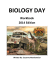More information

### Thank you, kindred inhabitants of earth.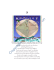More information

### Utilization of Base Model to create 3D Mobile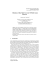More information

### art 2 – trompe l`oeil pencil drawing “fool the eye”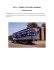More information

### The ELEMENTS of art - Mr Grimm Visual Arts Classes Vance High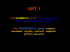More information

### Albino Animals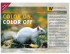More information

### Using Gigapixel Landscape Textures in Dragon Commander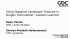More information

### Hans - Workshop - KC History Success.pptx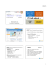More information

### 5 Use of reference materials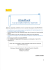More information

### Before you start editing textures, always create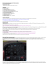More information

### Image Style Transfer Using Convolutional Neural Networks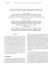More information

### REGU®-FADE - Cosmetics Online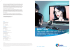More information

### A microrheological study of sickle hemoglobin polymerization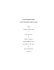More information